• 偏回归系数
万次阅读 多人点赞
2020-03-29 16:27:15

### 目录

假设有两个随机变量 ( x , y ) (x,y) ，其 N N 个样本组合为 （ x 1 , x 2 , … , x N ） （x_1,x_2,\dots,x_N） ( y 1 , y 2 , … , y N ) (y_1,y_2,\dots,y_N)

## 一、基础知识

单个变量 x x 的特征值为：
标准差（standard deviation）: σ x = ∑ i = 1 N ( x i − x ˉ ) 2 N \sigma_x=\sqrt{\frac{\sum_{i=1}^N(x_{i}-\bar{x})^2}{N}}
方差（variance）：标准差的平方，即 σ x 2 \sigma_x^2

变量 X X Y Y 的特征值为：协方差(covariance): σ x y = ∑ i = 1 N ( x i − x ˉ ) ( y i − y ˉ ) N \sigma_{xy}=\frac{\sum_{i=1}^N(x_{i}-\bar{x})(y_{i}-\bar{y})}{N}

## 二、回归系数与相关系数

假设存在回归方程： y = a x + ε y y=ax+\varepsilon_y ，其中 ε y \varepsilon_y 表示误差项。

### 1.定义

回归系数（regression coefficient）: 度量一个变量对另一个变量的线性影响大小。如，用 y y x x 进行线性回归，得到的 x x 的系数即为回归系数，记为 r y x r_{yx} 。在上式中，我们可知， r y x = a r_{yx}=a

相关系数（correction coefficient）: 也称作Pearson相关系数，用来度量两个变量之间的相关性（或联系的紧密程度）。该系数取值为 [ − 1 , 1 ] [-1,1] ，如果越靠近正负1，表明两个变量之间的线性关系越明显；越接近0，表明两个变量之间几乎没有线性关系。当其为0时，说明两个变量之间不存在线性关系。

### 2.二者的联系

回归系数 r r : 令 r y x r_{yx} 表示用 y y x x 作线性回归后得到的 x x 的回归系数，其计算方法为：
r y x = ∑ i = 1 N ( x i − x ˉ ) ( y i − y ˉ ) ∑ i = 1 N ( x i − x ˉ ) 2 = ∑ i = 1 N ( x i − x ˉ ) ( y i − y ˉ ) N ∑ i = 1 N ( x i − x ˉ ) 2 N = σ x y σ x 2 . ( 1 ) \begin{aligned} r_{yx}&=\frac{\sum_{i=1}^N(x_i-\bar{x})(y_i-\bar{y})}{\sum_{i=1}^N(x_i-\bar{x})^2}\\ &=\frac{\frac{\sum_{i=1}^N(x_i-\bar{x})(y_i-\bar{y})}{N}}{\frac{\sum_{i=1}^N(x_i-\bar{x})^2}{N}}\\ &=\frac{\sigma_{xy}}{\sigma_x^2}. \end{aligned}(1)
相关系数 ρ \rho

变量 y y x x 的相关系数的计算方法为：
ρ y x = ∑ i = 1 N ( x i − x ˉ ) ( y i − y ˉ ) ∑ i = 1 N ( x i − x ˉ ) 2 ∑ i = 1 N ( y i − y ˉ ) 2 = ∑ i = 1 N ( x i − x ˉ ) ( y i − y ˉ ) N ∑ i = 1 N ( x i − x ˉ ) 2 N ∑ i = 1 N ( y i − y ˉ ) 2 N = σ x y σ x σ y . ( 2 ) \begin{aligned} \rho_{yx}&=\frac{\sum_{i=1}^N(x_i-\bar{x})(y_i-\bar{y})}{\sqrt{\sum_{i=1}^N(x_i-\bar{x})^2}\sqrt{\sum_{i=1}^N(y_i-\bar{y})^2}}\\ &=\frac{\frac{\sum_{i=1}^N(x_i-\bar{x})(y_i-\bar{y})}{N}}{\sqrt{\frac{\sum_{i=1}^N(x_i-\bar{x})^2}{N}}\sqrt{\frac{\sum_{i=1}^N(y_i-\bar{y})^2}{N}}}\\ &=\frac{\sigma_{xy}}{\sigma_x\sigma_y}. \end{aligned}(2)
所以，由上面两个式子联立可得：
r y x = ρ y x ⋅ σ y σ x . r_{yx}=\rho_{yx}\cdot\frac{\sigma_y}{\sigma_x}.
类似地，拓展到多元线性回归的情况下，假设偏方差 σ y ⋅ z 2 \sigma_{y\cdot z}^2 表示固定 z z 的前提下 y y 的方差，则有偏回归系数 r y x ⋅ z r_{yx \cdot z} 和偏相关系数 ρ y x ⋅ z \rho_{yx \cdot z} 之间的关系为：
r y x ⋅ z = ρ y x ⋅ z ⋅ σ y ⋅ z σ x ⋅ z . r_{yx\cdot z}=\rho_{yx \cdot z}\cdot \frac{\sigma_{y\cdot z}}{\sigma_{x\cdot z}}.

### 3.二者的区别

（1）意义上：回归系数是描述自变量如何在数值上与因变量的相关性，即 r y x r_{yx} 表示 x x 每增（减）1个单位， y y 平均改变 a a 个单位；而相关系数是一种统计度量方法，用于度量变量之间的相关关系的密切程度。

（2）用途上：回归系数是为了拟合最佳模型，在已知另一个自变量的基础上预测对应的因变量；而相关系数是用来衡量变量之间的线性相关关系。

（3）对称性：用 x x y y 进行线性回归得到的回归系数 r x y r_{xy} 不等于用 y y x x 进行线性回归得到的回归系数 r y x r_{yx} ；而 x x y y 的相关系数 ρ x y \rho_{xy} 等于 y y x x 的相关系数 ρ y x \rho_{yx}

（4）变量含义：回归系数 r y x r_{yx} 蕴含了自变量 x x 的单位变化对因变量 y y 的影响；相关系数 ρ y x \rho_{yx} 表示自变量 x x 和因变量 y y 一起变化的程度。

（5）取值范围：回归系数的取值范围为 [ − ∞ , ∞ ] [-\infty,\infty] ，相关系数的取值范围为 [ − 1 , 1 ] [-1,1]

参考资料：

1. 线性回归，维基百科.
更多相关内容
• 对多元线性回归进行因子筛选，最后给出一定显著性水平下各因子均为显著的回归方程中的' 诸回归系数偏回归平方和、估计的标准偏差、复相关系数、F-检验值、各回归系数的标准' 偏差、应变量条件期望值的估计值及残差...
• 运用Python的数组和矩阵操作模拟验证一阶自回归模型中，自回归系数OLS估计量的有限样本偏差问题。
• 在前期内容中，我们介绍了如何对数据进行标准化处理，将...那么，提到不同变量的效应大小，大家一定会联想到在多因素回归模型中所得到的回归系数。例如，我们假设自变量分别为身高和体重，根据回归系数很容易就知...

在前期内容中，我们介绍了如何对数据进行标准化处理，将原始的连续型变量转化为一个无量纲的标准化数值，消除不同变量之间因性质、量纲、数量级等属性的差异而带来的影响，从而使不同变量的效应大小具有可比性，因此数据的标准化处理在实际的统计分析中也得到了较为广泛的应用。

那么，提到不同变量的效应大小，大家一定会联想到在多因素回归模型中所得到的回归系数。例如，我们假设自变量分别为身高和体重，根据回归系数很容易就知道每增加1cm的身高或每增加1kg的体重，引起的对因变量Y的影响大小，但是两者相比之下，到底谁的作用大谁的作用小呢？

原始的回归系数已经无法回答这样的问题，我们需要借助标准化回归系数来进行判断，今天我们就来向大家介绍一下，在回归模型中这个标准化回归系数到底是个什么鬼？

标准化回归系数

VS

未标准化回归系数

1、未标准化回归系数

通常我们在构建多因素回归模型时，方程中呈现的是未标准化回归系数，它是方程中不同自变量对应的原始的回归系数。它反映了在其他因素不变的情况下，该自变量每变化一个单位对因变量的作用大小。通过未标准化回归系数和常数项构建的方程，便可以对因变量进行预测，并得出结论。

2、标准化回归系数

而对于标准化回归系数，它是在对自变量和因变量同时进行标准化处理后所得到的回归系数，数据经过标准化处理后消除了量纲、数量级等差异的影响，使得不同变量之间具有可比性，因此可以用标准化回归系数来比较不同自变量对因变量的作用大小。

通常我们主要关注的是标准化回归系数的绝对值大小，绝对值越大，可认为它对因变量的影响就越大

3、两者的区别

未标准化回归系数体现的是自变量变化对因变量的绝对作用大小，而标准化回归系数反映的是不同自变量对因变量的相对作用大小，可以显示出不同自变量对因变量影响的重要性。

如果用标准化回归系数构建方程，得到的结论是有偏差的，因为此时自变量和因变量的数据都发生了转化，成为了标准化数据，因此标准化回归系数不能用于构建回归方程。

标准化回归系数

VS

每变化1个标准差的回归系数

我们在前期文章《回归模型中引入连续变量，还有哪些玩法？》中，介绍到对于连续型变量，在纳入多因素回归模型中时，可以将其转变为每变化1个标准差的形式，具体的操作方法是对原始的自变量进行标准化处理，然后再带入到回归模型中，所得到的回归系数即为该自变量每变化1个标准差对应的回归系数。

那么，我们假设此时有两个自变量，一个自变量的标准差为1，另一个自变量的标准差为100，两者对因变量都具有一定的影响。如果用上述每增加1个标准差对应的回归系数，来判断哪个自变量对因变量的影响更大的话，当同样变化1个标准差时，第一个自变量只需要改变1个单位，而第二个自变量则需要改变100个单位，因此标准差大的自变量改变起来就显得比较困难。此时，我们就需要用标准化回归系数来救场。

每增加1个标准差对应的回归系数，反映的是自变量每变化1个标准差时对因变量原始值变化产生的影响。而标准化回归系数，它反映的是自变量每变化1个标准差时，对因变量变化1个标准差产生的影响。

在计算每增加1个标准差对应的回归系数时，我们只需要对原始自变量进行标准化处理；而如果要计算标准化回归系数，则需要对原始的自变量和因变量同时进行标准化处理，标准化为标准正态分布对应的值后再构建回归模型。

SPSS操作

一、研究实例

某研究人员收集了100名研究对象的最大摄氧量(VO2 max)，并记录了他们的年龄、体重、心率等信息，拟探讨年龄、体重、心率对VO2 max的作用大小，同时评价上述哪一个因素对VO2 max的影响作用更大。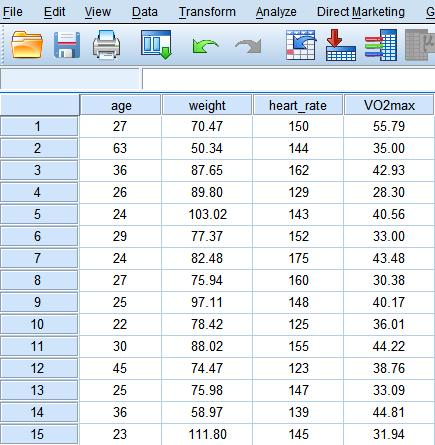二、操作步骤

1、多重线性回归

(操作步骤参考文章《SPSS实例教程：多重线性回归，你用对了么？》)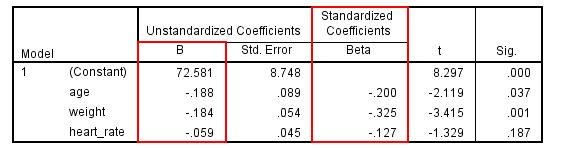根据多重线性回归分析的结果，回归方程可写为：

VO2 max = 72.581 – 0.188 * age – 0.184 * weight – 0.059 * heart_rate

其实不难发现，在SPSS的回归结果中，不仅展示了未标准化回归系数(Unstandardized Coefficients)，同时也得出了标准化回归系数(Standardized Coefficients)。

注意，未标准化回归系数更大的自变量，其标准化回归系数不一定更大。例如本例中，age的未标准化回归系数绝对值为0.188，大于weight的绝对值0.184，但是weight的标准化回归系数绝对值为0.325，却大于age的标准化回归系数绝对值0.200，说明weight对于VO2 max的影响较age更大。

2、数据标准化处理

(操作步骤参考前期推送文章《你听说过数据标准化处理吗？》)

虽然SPSS在回归结果中可以直接输出标准化回归系数，但为了加深对它的理解，我们可以亲自对数据进行一遍标准化处理，对上述结果进行一下验证。

Analyze → Descriptive Statistics → Descriptives → Save standardized values as variables

通过上述步骤分别生成4个标准化处理后的新变量。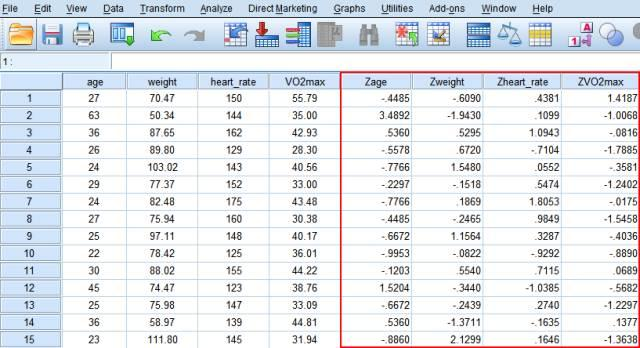3、标准化回归系数

在进行多重线性回归时，以VO2 max的标准化形式作为因变量，以age、 weight、 heart_rate的标准化形式作为自变量构建模型。结果发现，所得的结果与上述结果一致。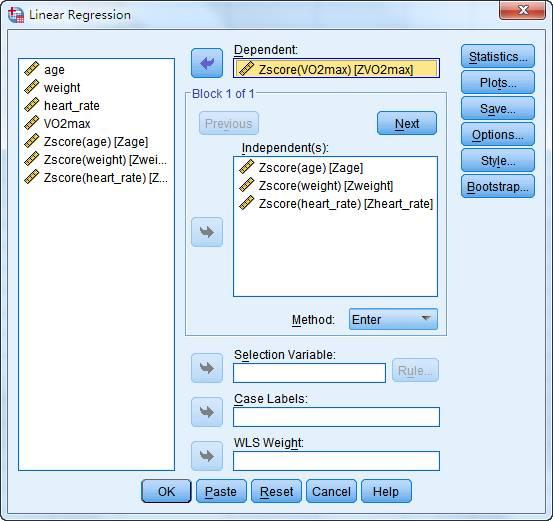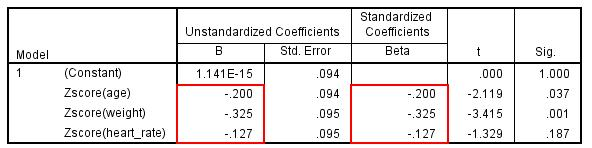相互转换关系

今天我们介绍了未标准化回归系数、每变化1个标准差的回归系数、以及标准化回归系数，最后再跟大家补充一下三者之间的相互转换关系。

每变化1个标准差的回归系数= 未标准化回归系数 * 该自变量的标准差

标准化回归系数= 未标准化回归系数 * 该自变量的标准差 / 因变量的标准差

大家可以根据上述转换关系自行进行验证哈。

哦，对了，细心的同学会发现，在SPSS中多重线性回归可以直接输出标准化回归系数，但是在logistic回归中，SPSS只能输出原始的未标准化的回归系数，如果我们想要计算logistic回归的标准化回归系数，比较不同自变量对因变量的相对作用大小，应该怎么办呢？

根据上述标准化回归系数的转换关系，在logistic回归中随机变量分布函数的标准差为π / √3 = 1.8138，故标准化回归系数 = 未标准化回归系数 * 该自变量的标准差 / 1.8138，即可计算logistic回归的标准化回归系数。

参考文献

 宋娜. 多元Logistic分布及其参数估计[D]. 北京工业大学, 2007.

医咖会微信：medieco-ykh

关注医咖会，及时获取最新统计教程

展开全文• Logistic回归虽然名字叫”回归” ，但却是一种分类学习方法。使用场景大概有两个：第一用来预测，第...并通过最小二乘法估计各个β系数的值。 如果y为二分类变量，只能取值0或1，那么线性回归方程就会遇到困难: 方程右

Logistic回归虽然名字叫”回归” ，但却是一种分类学习方法。使用场景大概有两个：第一用来预测，第二寻找因变量的影响因素。

# 一、从线性回归到Logistic回归

线性回归和Logistic回归都是广义线性模型的特例。

假设有一个因变量y和一组自变量x1, x2, x3, … , xn，其中y为连续变量，我们可以拟合一个线性方程：

y =β0 +β1x1 +β2x2 +β3x3 +…+βnxn

并通过最小二乘法估计各个β系数的值。

如果y为二分类变量，只能取值0或1，那么线性回归方程就会遇到困难: 方程右侧是一个连续的值，取值为负无穷到正无穷，而左侧只能取值[0,1]，无法对应。为了继续使用线性回归的思想，统计学家想到了一个变换方法，就是将方程右边的取值变换为[0,1]。最后选中了Logistic函数：

y = 1 / (1+e-x)

这是一个S型函数，值域为(0,1)，能将任何数值映射到(0,1)，且具有无限阶可导等优良数学性质。

我们将线性回归方程改写为：

y = 1 / (1+e-z),

其中，z =β0 +β1x1 +β2x2 +β3x3 +…+βnxn

此时方程两边的取值都在0和1之间。

进一步数学变换，可以写为：

Ln(y/(1-y)) =β0 +β1x1 +β2x2 +β3x3 +…+βnxn

Ln(y/(1-y))称为Logit变换。我们再将y视为y取值为1的概率p(y=1)，因此，1-y就是y取值为0的概率p(y=0)，所以上式改写为：

p(y=1) = ez/(1+ez),

p(y=0) = 1/(1+ez),

其中，z =β0 +β1x1 +β2x2 +β3x3 +…+βnxn.

接下来就可以使用”最大似然法”估计出各个系数β。

# 二、odds与OR复习

 odds: 称为几率、比值、比数，是指某事件发生的可能性(概率)与不发生的可能性（概率）之比。用p表示事件发生的概率，则：odds = p/(1-p)。

OR：比值比，为实验组的事件发生几率(odds1)/对照组的事件发生几率(odds2)。


# 三、Logistic回归结果的解读

 我们用一个例子来说明，这个例子中包含200名学生数据，包括1个自变量和4个自变量：

因变量:  hon，表示学生是否在荣誉班(honors class)，1表示是，0表示否；

自变量：

female ：性别，分类变量，1=女，0=男

write: 写作成绩，为连续变量

math：数学成绩，为连续变量

1、不包含任何变量的Logistic回归

首先拟合一个不包含任何变量的Logistic回归，

模型为 ln(p/(1-p) =β0

回归结果如下（结果经过编辑）：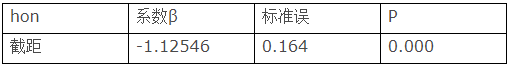hon取值为1的概率p为49/(151+49) = 24.5% = 0.245，我们可以手动计算出ln(p/(1-p) = -1.12546，等于系数β0。可以得出关系：

β0=ln(odds)。

2、包含一个二分类因变量的模型

拟合一个包含二分类因变量female的Logistic回归，

模型为 ln(p/(1-p)  =β0 +β1* female.

回归结果如下（结果经过编辑）：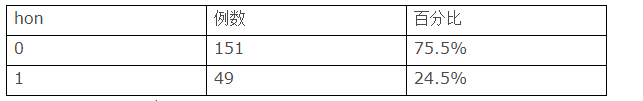在解读这个结果之前，先看一下hon和female的交叉表：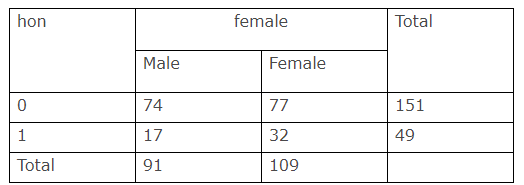3、包含一个连续变量的模型根据这个交叉表，
对于男性（Male），其处在荣誉班级的概率为17/91，

处在非荣誉班级的概率为74/91，所以其处在荣誉班级的几率odds1=(17/91)/(74/91) = 17/74 = 0.23；

相应的，女性处于荣誉班级的几率odds2 = (32/109)/(77/109)=32/77 = 0.42。

女性对男性的几率之比OR = odds2/odds1 = 0.42/0.23 = 1.809。

我们可以说，女性比男性在荣誉班的几率高80.9%。

回到Logistic回归结果。

截距的系数-1.47是男性odds的对数（因为男性用female=0表示，是对照组），ln(0.23) = -1.47。

变量female的系数为0.593，是女性对男性的OR值的对数，ln(1.809) = 0.593。

所以我们可以得出关系: OR = exp(β)，或者β= ln(OR)（exp(x)函数为指数函数，代表e的x次方）。

3、包含一个连续变量的模型

拟合一个包含连续变量math的Logistic回归，

模型为 ln(p/(1-p)  =β0 +β1* math.

回归结果如下（结果经过编辑）：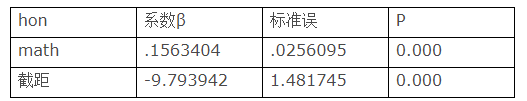这里截距系数的含义是在荣誉班中math成绩为0的odds的对数。我们计算出odds = exp(-9.793942) = .00005579，是非常小的。因为在我们的数据中，没有math成绩为0的学生，所以这是一个外推出来的假想值。

怎么解释math的系数呢？根据拟合的模型，有：

ln(p/(1-p)) =  - 9.793942  + .1563404*math

我们先假设math=54，有：

ln(p/(1-p))(math=54) = - 9.793942 + .1563404 *54

然后我们把math提高提高一个单位，令math=55，有：

ln(p/(1-p))(math=55) = - 9.793942 + .1563404 *55

两者之差：

ln(p/(1-p))(math=55) - ln(p/1-p))(math = 54) = 0.1563404.

正好是变量math的系数。

由此我们可以说，math每提高1个单位，odds（即p/(1-p)，也即处于荣誉班的几率）的对数增加0.1563404。

那么odds增加多少呢？根据对数公式：

ln(p/(1-p))(math=55) - ln(p/1-p))(math = 54) = ln((p/(1-p)(math=55)/ (p/(1-p)(math=54))) = ln(odds(math=55)/ odds(math=54)) = 0.1563404.

所以：

odds(math=55)/ odds(math=54)  =  exp(0.1563404) = 1.169.

因此我们可以说，math每升高一个单位，odds增加16.9%。且与math的所处的绝对值无关。

聪明的读者肯定发现，odds(math=55)/ odds(math=54)不就是OR嘛！

4、包含多个变量的模型（无交互效应）

模型为 ln(p/(1-p) = β0 +β1* math+β2* female+β3* read.

回归结果如下（结果经过编辑）：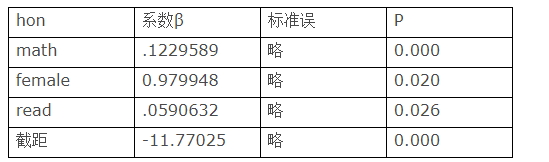该结果说明:

5、包含交互相应的模型

拟合一个包含female、math和两者交互相应的Logistic回归，

模型为 ln(p/(1-p)  =β0 +β1* female+β2* math+β3* female *math.

所谓交互效应，是指一个变量对结果的影响因另一个变量取值的不同而不同。

回归结果如下（结果经过编辑）：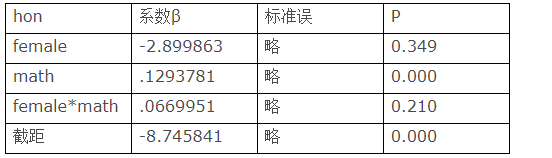注意：female*math项的P为0.21，可以认为没有交互相应。但这里我们为了讲解交互效应，暂时忽略P值，姑且认为他们是存在交互效应的。

由于交互效应的存在，我们就不能说在保持math和female*math不变的情况下，female的影响如何如何，因为math和female*math是不可能保持不变的!

对于这种简单的情况，我们可以分别拟合两个方程，

对于男性（female=0）：

log(p/(1-p))= β0 + β2*math.

对于女性（female=1）：

log(p/(1-p))= (β0 + β1) + (β2 + β3 )*math.

然后分别解释。


注：本文大量参考这篇文章：http://www.ats.ucla.edu/stat/mult_pkg/faq/general/odds_ratio.htm

展开全文hadoop 深度学习 数据挖掘 机器学习
• 直线回归系数的假设检验 对B=0进行假设检验 我们以下题数据为例作出解答： 并计算有关指标：（其中lxx、lxy为离均差平方和，lxy为离均差积和） 下面作出假设检验 并得出方差分析表 若用T检验 此外，我们另外...

## 直线回归系数的假设检验

对B=0进行假设检验我们以下题数据为例作出解答：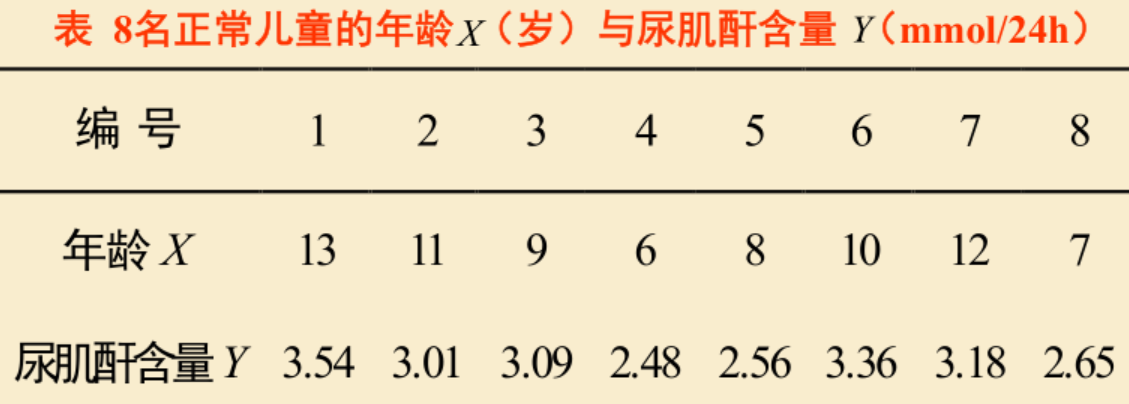并计算有关指标：（其中lxx、lxy为离均差平方和，lxy为离均差积和）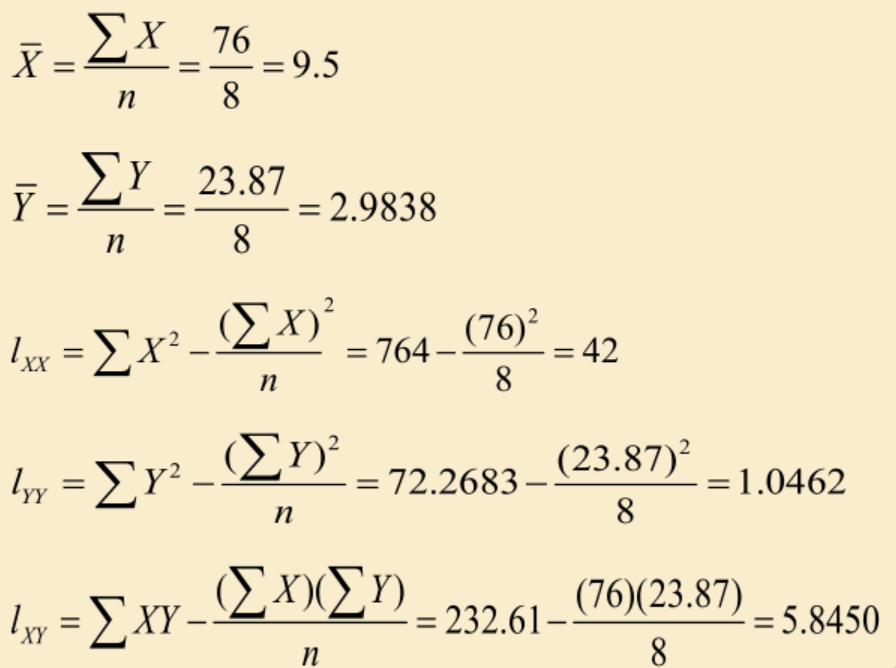下面作出假设检验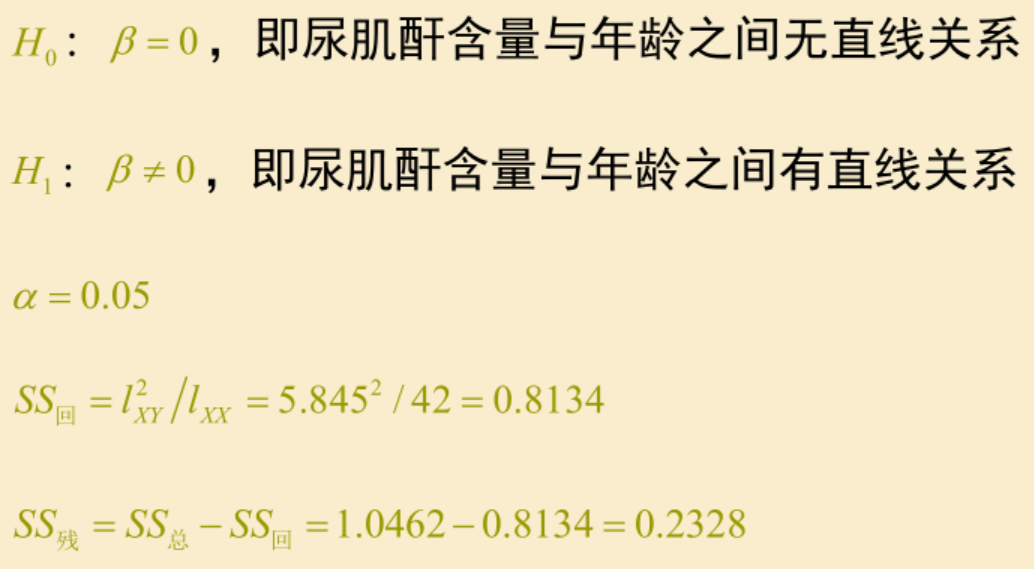并得出方差分析表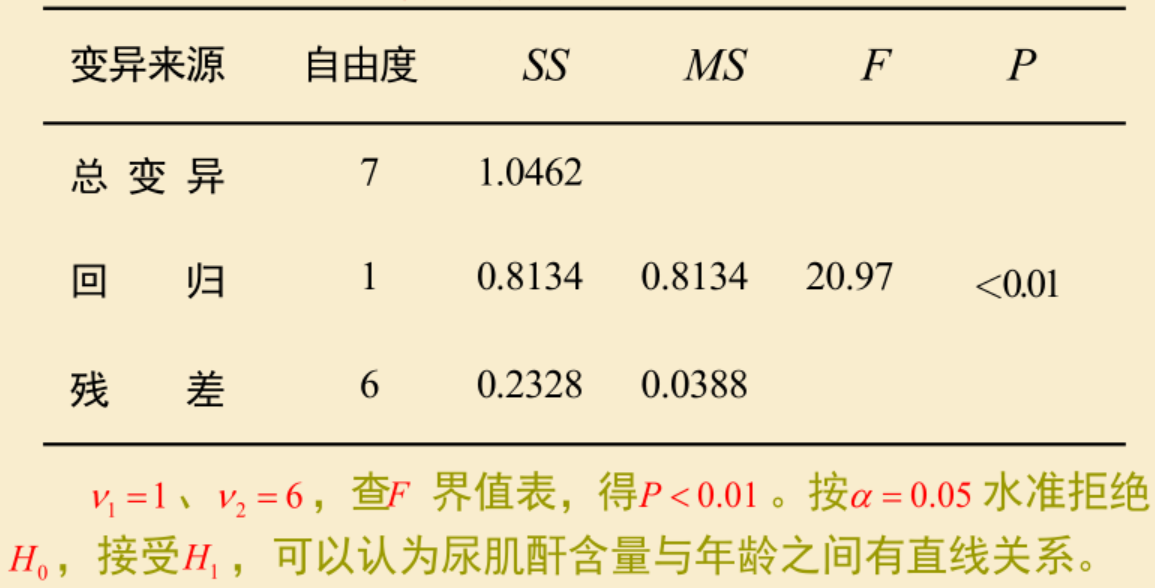若用T检验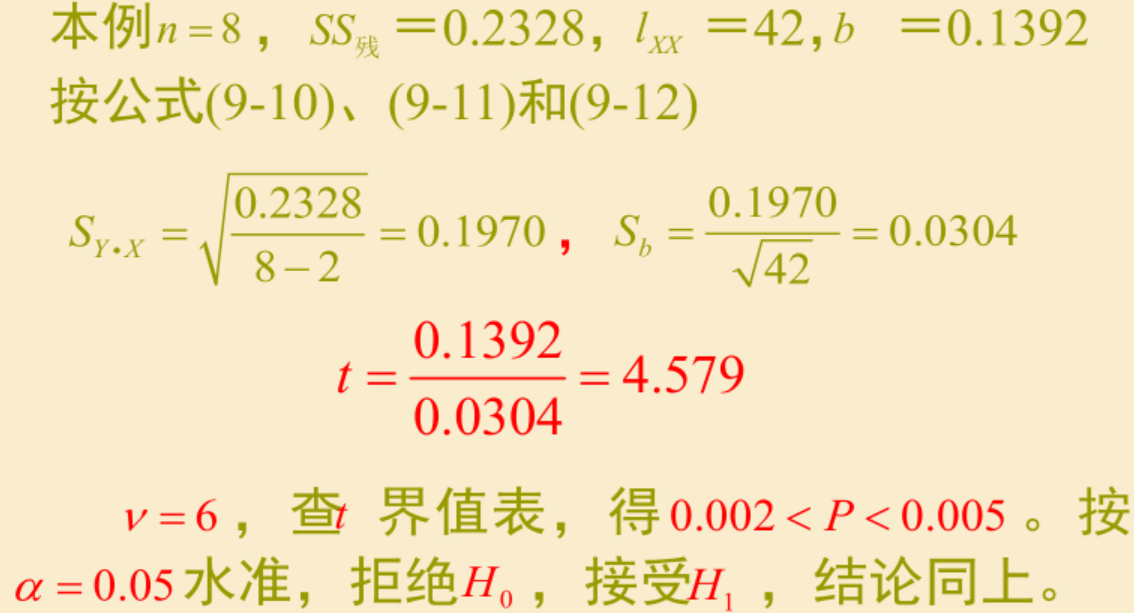此外，我们另外说明
反应两变量关系密切程度或数量上影响大小的统计量应该是回归系数相关系数的绝对值，而不是假设检验的P值。假设检验的P值越小只能说越有理由认为变量间的直线关系存在，而不能说关系越密切或越“显著”。另外，直线回归用于预测时，其适用范围一般不应超出样本中自变量的取值范围.

## 多元线性回归模型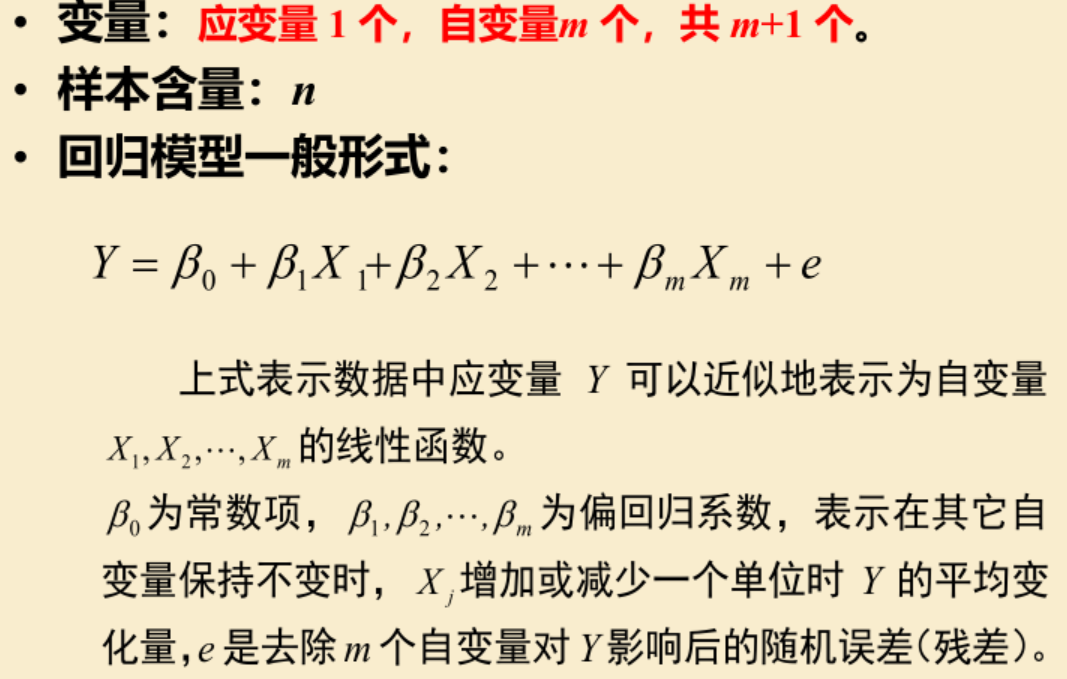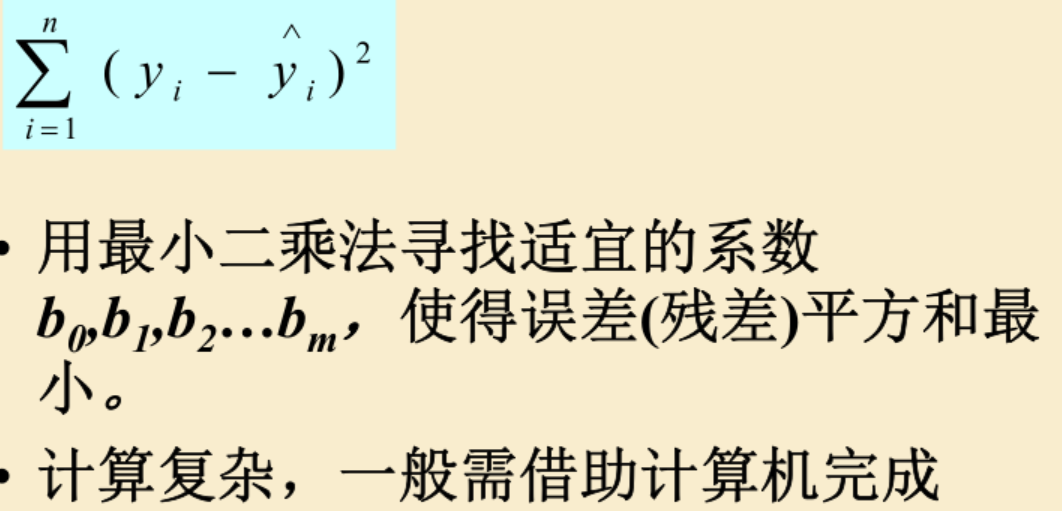我们可以通过SPSS统计软件进行计算。
对于假设检验分析，我们仍旧可以建立一个服从F分布的F统计量进行分析。这个统计量把总的离差平方和分解为两部分。一部分是由回归造成的平方和，一部分是由残差造成的平方和。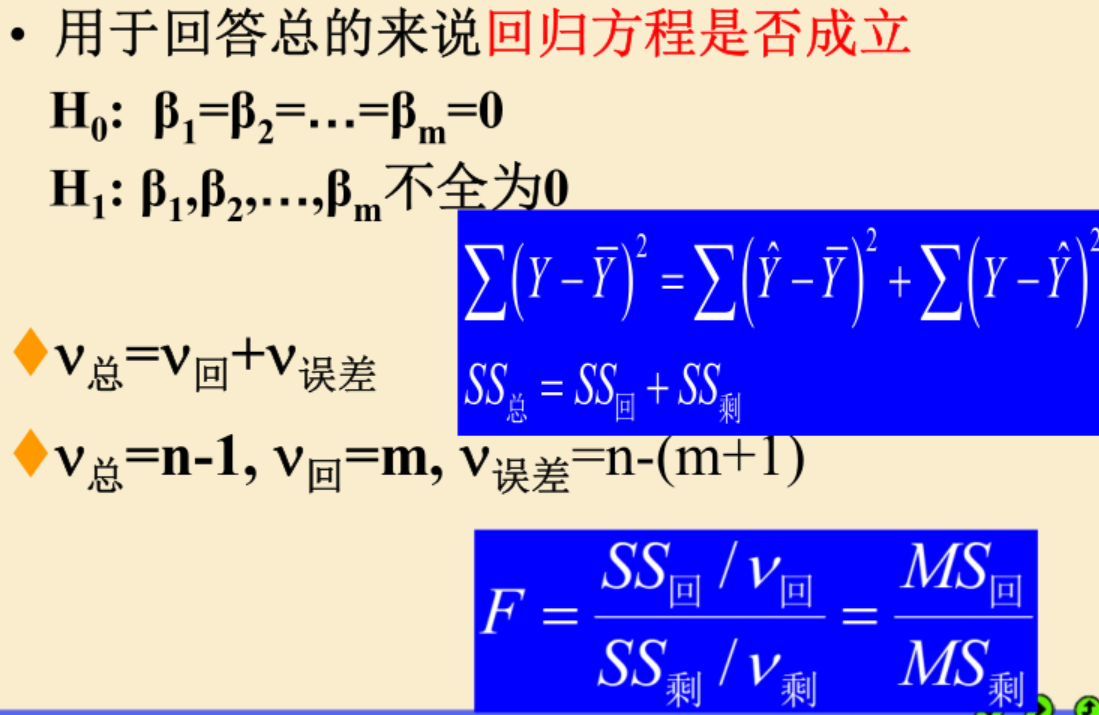regression为回归平方和，residual为残差平方和，total为总的平方和。因为有三个自变量，所以第一行df为3，且总数据有19个。sig为p值，是一个很小的数，所以这时候我们可以拒绝H0，认为回归方程是成立的。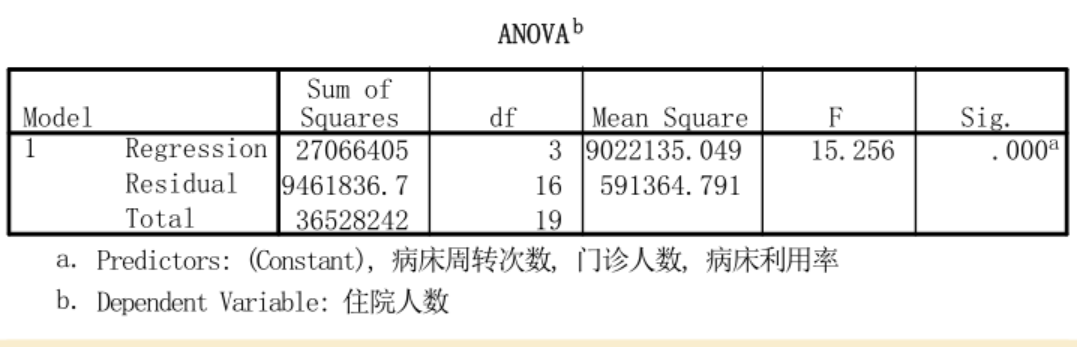但是回归方程有统计学意义并不说明每一个偏回归系数都有意义。
我们用T检验得到如下结果：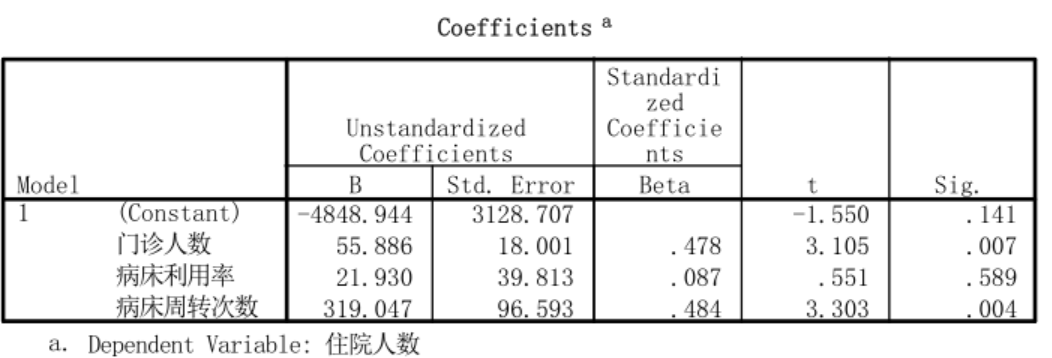此时看表可看出x1的p值为0.007，x3的p值为0.004.则可说明x1x3有显著意义，则要拒绝原假设。
从Beta可看出每个因子的影响，值越大影响越大

### 评价拟合效果指标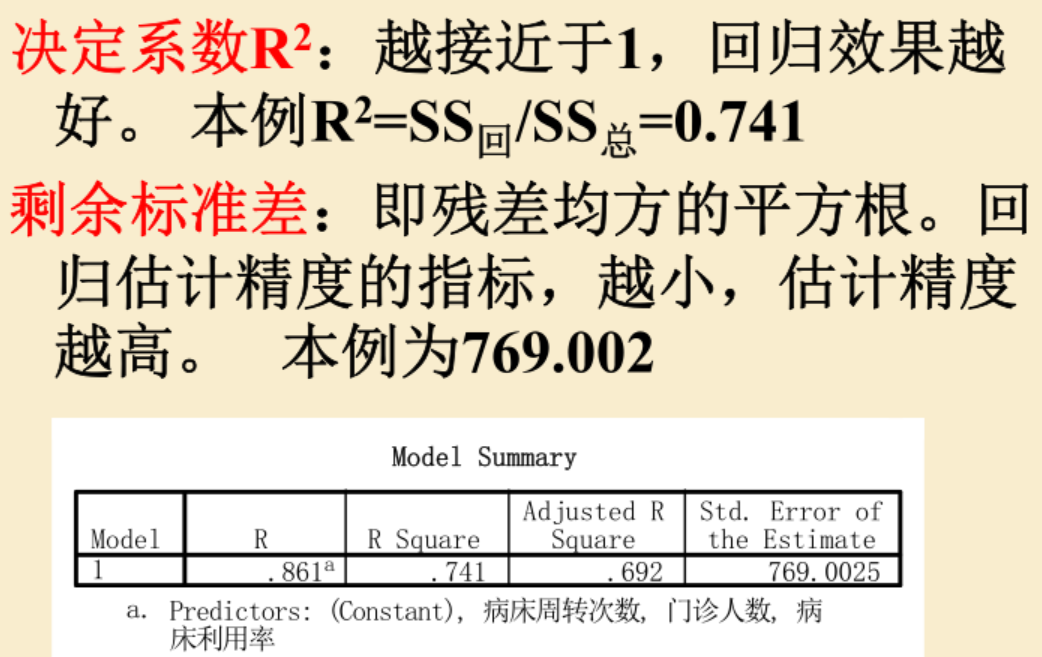R方为回归所造成的离差平方和和总的离差平方和的比值。R方越大，拟合越好。

展开全文• 数据回归-线性回归模型系数估计的研究和应用.pdf
• R语言相关或者部分相关性系数计算实战：使用psych包计算（Partial Correlation）相关系数、拟合回归模型使用两个回归模型的残差计算相关性系数 目录 R语言相关或者部分相关性系数计算实战：使用psych包...r语言 数据挖掘 机器学习 人工智能 自然语言处理
• 标准化的回归系数是指将数据标准化（减均值除方差）后计算得到的回归系数。因为数据进行了标准化，因此就取消了量纲的影响。假设因变量为y，自变量为x，标准回归系数为a。那么在解释时就要说，当x变化1个标准差是，y...标准化
• 数据回归-线性模型回归系数的一种有估计——综合C-K岭估计.pdf
• 数据回归-一般平衡损失下回归系数的最优线性无估计及可容许估计.pdf
• 在线性回归模型Y= Xβ+ε;E(ε) = 0;Cov(ε) =σ2V,V> 0下,给出了一种新的有估计-β(F(K) ) = (X′V-1X+ TF(K) T′)-1X′V-1Y,讨论了这种有估计的优良性,推广了已有的相关结果.
• 回归方程及回归系数的显著性检验.pdf回归方程及回归系数的显著性检验.pdf回归方程及回归系数的显著性检验.pdf回归方程及回归系数的显著性检验.pdf回归方程及回归系数的显著性检验.pdf回归方程及回归系数的显著性检验...
• 文章目录 十一、回归方程与回归系数的显著性检验 1.平方和分解 2.回归方程的假设检验 3.中心化的等价形式 4.回归系数的假设检验 5.回归方程的预报精度 回顾总结 十一、回归方程与回归系数的显著性检验 回归方程的...假设检验r语言 数据
• Author:龙箬 ...多元相关与回归分析及R使用 1.变量间的关系分析 简单相关分析的R计算 > x1=c(171,175,159,155,152,158,154,164,168,166,159,164) #身高 > x2=c(57,64,41,38,35,44,41,51,57,49.r语言
• 提出了齐次等式约束线性回归模型回归系数的一个新的有估计，即综合条件岭估计．讨论了综合条件岭估计的可容许性等优良性质．给出了其迭代解和极小化均方误差的无估计解．在一定的条件下，综合条件岭估计的样本总...
• 缺失t正态数据下线性回归系数的稳健估计，黄亚慧，钟波，本文研究缺失t正态数据下线性回归模型系数的稳健估计问题。对于存在缺失的t正态分布数据，运用最小一乘方法估计线性回归模型�
• ## 偏相关系数

万次阅读 2020-03-23 20:58:56
文章目录相关分析模型相关系数的计算1.一阶相关系数计算2.二阶相关系数计算相关系数的假设检验 相关分析模型 当研究某一种因素对另一种因素的影响或相关程度，把其他因素的影响排除在外，而单独研究这...
• 1、最小二乘法(min square)求解回归系数 将训练特征表示为 X 矩阵，结果表示成 y 向量，仍然是线性回归模型，误差函数不变。那么 θ 可以直接由下面公式得出 用矩阵可表示为 因为要求函数的极小值，对θ求导...最小二乘法 梯度下降法
• 针对引起线性回归模型LS估计性能变坏的根本原因,提出了回归系数的广义c-K估计,将众多经典的有估计结合在一起,对有估计的改进进行了研究,分别证明了最小化均方误差和数量化矩阵K均可对Stein估计进行改进,给出了...
• １、回归方程的显著性检验(1) 回归平方和与剩余平方和建立回归方程以后, 回归效果如何呢？因变量与自变量是否确实存在线性关系呢？这是需要进行统计检验才能加以肯定或否定, 为此, 我们要进一步研究因变量取值的变化...
• 2.2 多元线性回归系数的图形解释 我们运用 Stata 自带的 1978 汽车数据集 auto.dta 中的进口汽车 (foreign) 数据来解释多元回归系数估计值的含义。 首先，我们把汽车的百英里油耗 (100/mpg) 当作被响应变量，汽车的...
• 回归系数的解释 yi=β0+β1x1i+β2x2i+⋯+βkxki+μiyi=β0^+β2^x2i+⋯+βk^xkiβ0^的数值意义一般不考虑，因为所有的自变量一般不会同时全为0.βm^(m=1,2,⋯ ,k):控制其他自变量不变的情况下，xmi每增加一个单位...python 大数据 算法
• ## 偏回归

千次阅读 2014-07-15 17:03:23
偏相关系数 Partial correlation coefficient 在多元回归分析中，在消除其他...偏回归系数 partial regression coefficient 在多元回归分析中，随机因变量对各个自变量的回归系数，表示各自变量对随机变量的影响程度
• ## 多元线性回归

万次阅读 2016-04-14 11:57:54
假定被解释变量与多个解释变量之间具有线性关系，是解释变量的多元线性函数，称为多元线性回归模型。即  (1.1) 其中为被解释变量，为个解释变量，为个未知参数，为随机误差项。 被解释变量的期望值与解释...
• SPSS（六）SPSS之回归分析衍生方法（图文+数据集） 我们知道线性回归是有适用条件的 因变量的独立性 正态性 方差齐性 无极端值 自变量、因变量要有线性趋势 假如不满足以上的条件，还能做回归分析吗？其实有...曲线拟合过程
• 目录 1. 简介 2.... 4.1 使用 qreg 的简单条件分位数回归 4.2 使用 bsqreg 估计 4.3 使用 qrprocess 估计 4.4 使用 qreg2 估计 4.5 使用 ivqreg2 估计 4.6 使用 xtqreqstata绘图 连享会
• 目的: 测试单变量与目标变量的线性关系...求相关系数的sas 实现： ods graphics on; proc corr data=fish1 plots=scatter(alpha=0.2 0.3); var height weight; partial length weight; run; ods graphics off;...